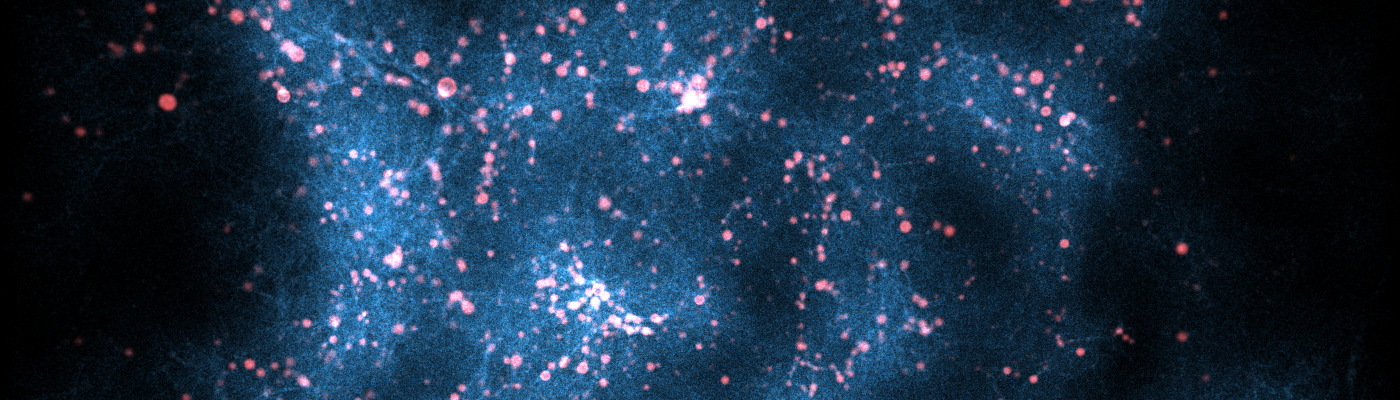# Introduction

Lyman-$\alpha$ spectra can provide insights into the small-scale structure and kinematics of neutral hydrogen (HI) within galaxies as well as the ionization state of the intergalactic medium (IGM). The former defines the intrinsic spectrum of a galaxy, which is modified by the latter. These two effects are degenerate: While at low redshifts, double peaked Lyman-$\alpha$ spectra are the norm, we find more and more spectra only showing a red peak at high redshifts. Does this reflect imprints of larger outflow velocities on the galaxy scale or is this rather just an attenuation effect of increasing neutral hydrogen density of the IGM voiding flux bluewards of the Lyman-$\alpha$ line-center? Are there certain spectral features imprinted by the IGM break this degeneracy?

# Approach

We investigate the IGM impact on Ly$\alpha$ spectral shapes using the IllustrisTNG100 simulations between redshifts $0$ to $5$, where we integrate the Ly$\alpha$ optical depth

$$\tau(\lambda_{\mathrm{i}}) = \int_{s_0}^\infty \mathrm{d}s \ n_\mathrm{HI}(s)\ \sigma\left(\lambda(\lambda_{\mathrm{i}},v,s),T_{\rm HI} \right)$$

for a range of input wavelengths $\lambda_i$ starting at some $s_0$ outside of the galaxy, typically $1.5$ times the virial radius of the host halo.

Calculating the optical depth this way for a large set of lines of sight, and halos as potential sites of Lyman-$\alpha$ emitting galaxies, gives us a catalogue of transmission curves for our further analysis.

As a starting point, we use simplified Lyman-$\alpha$ spectra that might arise within the galaxies/halos to convolve with these transmission curves. This convolution yields mock spectra, which we can statistically analyse for variations arising due to the IGM interaction. We can show that the distributions of peak counts and asymmetry in the spectra can lift the degeneracy between the intrinsic spectrum and the IGM absorption. See our study published here for more details and results.Spectrum and IGM transmission of a simulated Lyman-$\alpha$ emitting galaxy at $z=3.0$ in IllustrisTNG100. In solid red, we show the resulting spectrum in a full radiative transfer (RT) simulation and alternatively without the IGM (red dotted). Complementary, we plotted one transmission curve we computed for our study for the chosen line of sight. Multiplying the transmission curve with the full radiative transfer simulation without IGM, we find that we can reconstruct all spectral imprints of the IGM without the need for a full RT simulation.

# Public Data Release

We make a reasonably sized subset of our transmission curves available here. See below for a simple example how to load and use this data set in python.##### Chris Byrohl
###### PhD Student

My research interests include Lyman-$\alpha$ radiation to study galaxies and the large-scale structure, supernovae type Ia and high-performance computing.